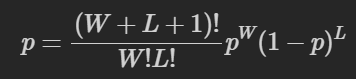Just realised that the Obsidian text editor supports MathJax.

So for the mathematically inclined, write your Latex equations either inline by surrounding them with $s, or on their own line starting with $$. It’ll transform e.g.: $$ p = \frac{(W+L+1)!}{W!L!} p^W(1-p)^L$\$

into: